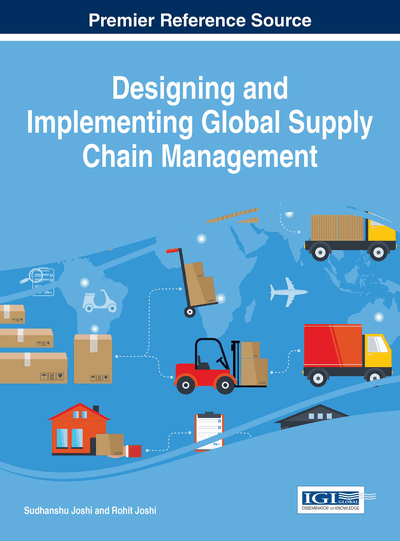# Intuitionistic Fuzzy Sets-Based Method for Multi-Criteria Decision-Making

Bhagawati P. Joshi (Seemant Institute of Technology, India) and Sanjay Kumar (G. B. Pant University of Agriculture and Technology, India)
Copyright: © 2016 |Pages: 14
DOI: 10.4018/978-1-4666-9720-1.ch002
OnDemand PDF Download:
Available
\$37.50
No Current Special Offers

## Abstract

In this study, the familiarity or expertise of the experts with the evaluation areas (called confidence levels) is also incorporated in the multi-criteria decision making (MCDM) process under intuitionistic fuzzy environment, i.e. two types of information is involved: one is the performance of the evaluation objects under intuitionistic fuzzy environment and other is the familiarity or expertise with the evaluation areas. In this direction recently, Yu (2014) proposed some operators to aggregate the two types information described above effectively. In this paper, the weighted confidence level is used instead of simply confidence levels as used by Yu (2014) to enhance MCDM method under intuitionistic fuzzy environment. So, two operators such as intuitionistic fuzzy weighted averaging operator under weighted confidence level (IFWAOUWCL) and intuitionistic fuzzy weighted geometric operator under weighted confidence level (IFWGOUWCL) are introduced to aggregate the two types of information. Finally, the presented technique is implemented effectively to a real life problem of supply selection.
Chapter Preview
Top

## Introduction

Zadeh (1965) introduced the concept of fuzzy sets (FSs) as the generalization of traditional classical sets. Atanassov (1983, 1986) introduced the concept of intuitionistic fuzzy set (IFS) as a generalization of FSs, which is characterized by a membership degree, non-membership degree, and a hesitancy degree. The theory of IFS received more and more attention due to the capability of dealing with imprecise information. Gau and Buehrer (1993) introduced the concept of vague set. However, Bustine and Burillo (1996) proved that the notion of vague set is the same as that of IFS. The IFS theory is used to deal with many real life problems. Many researchers (Xu, 2007; Xu, 2007) successfully employed the concept of IFS theory in intuitionistic fuzzy multi-criteria group decision-making (IFMCDM) problems. The aggregation of intuitionistic fuzzy values (IFVs) is a very important part in IFMCDM problems. So, the aggregation operators play an important role during the aggregation of information in IFMCDM problems. In this direction many researchers are interested in defying different types of aggregation operators. Xu (2007) and Xu and Yager (2006) introduced the intuitionistic fuzzy weighted averaging (IFWA) operator and intuitionistic fuzzy weighted geometric (IFWG) operator respectively.

Zhao et. al. (2010) introduced intuitionistic fuzzy ordered weighted averaging (IFOWA) operator, intuitionistic fuzzy ordered weighted geometric (IFOWG) operator, intuitionistic fuzzy hybrid averaging (IFHA) operator, intuitionistic fuzzy hybrid geometric (IFHG) operator, generalized IFWA, generalized IFWG, generalized IFOWA and generalized IFOWG. Xu and Wang (2012) presented the induced generalized aggregation operators for intuitionistic fuzzy sets. Xia et. al. (2013) introduced intuitionistic multiplicative weighted averaging (IMWA) operator, intuitionistic multiplicative weighted geometric (IMWG) operator, generalized IMWA operator and generalized IMWG operator to aggregate intuitionistic fuzzy preference information. Xia and Xu (2013) presented group decision making method based on intuitionistic multiplicative aggregation operators.

To the aggregation of intuitionistic fuzzy information, many of the existing aggregation operators do not mention the confidence level of the information provided by the experts. Xia et. al. (2011) proposed a series of aggregation operators considering the confidence levels of the aggregated arguments. Yu (2013) presented some generalized belief aggregation operators to aggregate the IFVs. Recently, Yu (2014) proposed some operators under the confidences levels such as confidence intuitionistic fuzzy aggregation operator, confidence intuitionistic fuzzy ordered aggregation operator and many more to deal with multi-criteria group decision making problems. In this paper, the weighted confidence level is used instead of simply confidence levels as used by Yu (2014) to the betterment of MCDM process under intuitionistic fuzzy environment. So, two operators such as intuitionistic fuzzy weighted averaging operator under weighted confidence level (IFWAOUWCL) and intuitionistic fuzzy weighted geometric operator under weighted confidence level (IFWGOUWCL) are introduced to aggregate the two types of information. Finally, the presented technique is implemented effectively to a real life problem of supply selection.

Top

## Background

In this section we briefly presented the necessary definitions and techniques to develop the present study.

## Complete Chapter List

Search this Book:
Reset